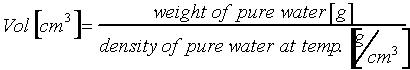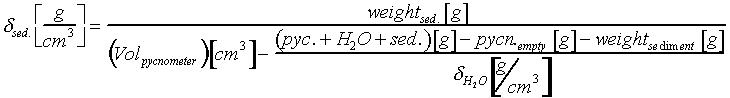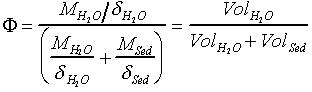## Methods / Description Density and Porosity

APPARATUS:
Analytical balance
Thermometer
Pycnometer, with exactly determined volume

HOW TO DETERMINE THE VOLUME OF THE PYCNOMETERS:
Leave the pycnometer and the pure water for several hours in the same room with the analytical balance. Weigh the empty, dry pycnometer. Then fill the pycnometer completely, without any air bubbles. Remove all drops on the outside and weigh again. From the difference between the two weights you get the weight of the pure water. Now calculate the volume of the pycnometer with the following formula:You find the densitiy of pure water at different temperatures in special tables (i.e. table 1.)

table 1.
T [°C]         density [g/cm3]
20                 0.998234
21                 0.998022
22                 0.997801
23                 0.997569
24                 0.997327
25                 0.997075
26                 0.996814
.

DETERMINATION OF DENSITY IN LIQUID SAMPLES
Leave the pycnometer and the samples for several hours in the same room with the analytical balance. Fill the pycnometer with the sample (as described before), weigh it and calculate the density with the following formula:

DETERMINATION OF DENSITY IN SOLID SAMPLES (i.e. SEDIMENTS)
Leave the pycnometer the pure water and the samples for several hours in the same room with the analytical balance. Weigh the empty, dry pycnometer.
Then fill the pycnometer completely with pure water, without any air bubbles. Remove all drops on the outside and weigh again. Repeat this for every sample.
Weigh in the dry, homogeneous sediment on a watch glass (1/8 of the pycnometer volume) and fill it carefully into the empty pycnometer. Fill up with pure water (without air bubbles), remove all drops on the outside and weigh again.

CALCULATION :d sed.=  density of the dry mass
weightsed.  =  weight of the sediment
VolPyknometer  =  volume of the pycnometer (determination as mentioned before)
(Pyc. +H2O+sed.) =  weight of the pycnometer, filled with sediment and pure water
Pycnleer  =  weight of empty, dry pycnometer
d H2O=  density of pure water at temperature (table 1.)

POROSITY:
The porosity is the volume fraction (0-1) of water in the sediment. This value is determined by drying the sediment. Wet sediment has to be weighed, lyophilizied and weighed again. From the difference of the two weights you can calculate the mass fraction (0-1), the dry mass and the content of H2O.f =  volumeparts of water in the sediment [0-1]
MH2O =  massfraction H2O (%)
MSed. =  massfraction dry mass (%)
dH2O =  density of pure water at temperature (g/cm3) (tab.1)
dsed.= density of the sediment (g/cm3)